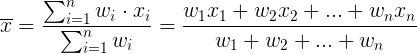# Average Calculator

* SD = standard deviation

Weighted average calculator ►

## Average calculation

So The average (arithmetic mean) is equal to the sum of the n numbers divided by [n].

Average = sum / count = (a1+a2+...+an) / n

#### Example 1

The average of 1,4,5 is:

Average = (1+4+5) / 3 = 10/3 = 3.3333333333

#### Example 2

The average of 1,4,9 is:

Average = (1+4+9) / 3 = 14/3 = 4.6666666667

#### Example 3

The average of 3,4,9 is:

Average = (3+4+9) / 3 = 16/3 = 5.3333333333

## Weighted average calculator

 Weight Data value

## Weighted average calculation

The weighted average (x) is equal to the sum of the product of the weight (wi) times the data number (xi) divided by the sum of the weights:#### Example 1

Find the weighted average of class grades (with equal weight) 50,50,80,80,80,90:

Since the weight of all grades are equal, we can calculate these grades with simple average or we can cound how many times each grade apear and use weighted average.

x = (50+50+80+80+80+90) / 6 = 430/6 = 71.666666667

#### Example 2

Find the weighted average of class grades (with equal weight) 50,50,70,70,80,90:

Since the weight of all grades are equal, we can calculate these grades with simple average or we can cound how many times each grade apear and use weighted average.

x  = (50+50+70+70+80+90) / 6 = 410/6 = 68.333333333

#### Example 3

Find the weighted average of class grades (with equal weight) 50,50,90,90,80,90:

Since the weight of all grades are equal, we can calculate these grades with simple average or we can cound how many times each grade apear and use weighted average.

x = (50+50+90+90+80+90) / 6 = 450/6 = 75

Weighted average calculator ►

## Features of Average Calculator

Our Average Calculator allows the users to Calculate Average. Some of the prominent features of this utility are explained below.

#### No Registration

You don’t need to go through any registration process to use the Average Calculator. Using this utility, users to Calculate Average as many times as you want for free.

#### Fast conversion

This Average Calculator offers users the fastest Calculate. Once the user enters the  Average values ​​in the input field and clicks the Calculate button, the utility will start the conversion process and return the results immediately.

#### Saves Time and Effort

The manual procedure of Calculator Average isn’t an easy task. You must spend a lot of time and effort to complete this task. The Average Calculator allows you to complete the same task immediately. You will not be asked to follow manual procedures, as its automated algorithms will do the work for you.

##### Accuracy

Despite investing time and effort in manual Calculation, you might not be able to get your hands on accurate results. Not everyone is good at solving math problems, even if you think you're a pro, there's still a good chance you'll get in accurate results. This situation can be smartly handled with the help of a Average Calculator. You will be provided with 100% accurate results by this online tool.

##### Compatibility

The online Average converter perfectly works on all operating systems. Whether you have a Mac, iOS, Android, Windows, or Linux device, you can easily use this online Tool without facing any hassle.

###### 100% Free

You don't need to go through any registration process to use this Average Calculator. You can use this utility for free and do unlimited Average Calculate without any limitations.